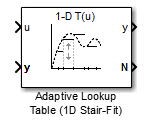# Adaptive Lookup Table (1D Stair-Fit)

## Library

•## Description

The Adaptive Lookup Table (1D Stair-Fit) block creates a one-dimensional adaptive lookup table by dynamically updating the underlying lookup table. The block uses the outputs, y, of your system to do the adaptations.

Each indexing parameter u may take a value within a set of adapting data points, which are called breakpoints. Two breakpoints in each dimension define a cell. The set of all breakpoints in one of the dimensions defines a grid. In the one-dimensional case, each cell has two breakpoints, and the cell is a line segment.

You can use the Adaptive Lookup Table (1D Stair Fit) block to model time-varying systems with one input.

Doubles only

## Parameters

First input (row) breakpoint set

The vector of values containing possible block input values. The input vector must be monotonically increasing.

Make initial table an input

Selecting this check box forces the Adaptive Lookup Table (1D Stair-Fit) block to ignore the Table data (initial) parameter, and creates a new input port `Tin`. Use this port to input the table data.

Table data (initial)

The initial table output values. This vector must be of size N-1, where N is the number of breakpoints.

Table numbering data

Number values assigned to cells. This vector must be the same size as the table data vector, and each value must be unique.

Select one of the following options:

• `Sample mean` — Average all the values received within a cell.

• `Sample mean (with forgetting)` — Give more weight to the new data. The weighting is determined by the Adaptation gain parameter.

A number between 0 and 1 that regulates the weight given to new data during the adaptation. A 0 means short memory (last data becomes the table value), and 1 means long memory (average all data received in a cell).

Selecting this check box creates an additional output port `Tout` for the adapted table.

Selecting this check box creates an additional input port `Enable` that enables, disables, or resets the adaptive lookup table. A signal value of `0` applied to the port disables the adaptation, and signal value of `1` enables the adaptation. Setting the signal value to `2` resets the table values to the initial table data.

Selecting this check box creates an additional input port `Lock` that provides the means for updating only specified cells during a simulation run. A signal value of `0` unlocks the specified cells and signal value of `1` locks the specified cells.

Action for out-of-range input

`Ignore` or `Adapt` by extrapolating beyond the extreme breakpoints.

## Version History

Introduced in R2009a